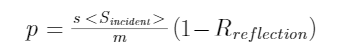# Force is equivalent to Power

Keying  Guan

Department of Mathematics, School of Science, Beijing Jiaotong University

Email: keying.guan@gmail.com

Just as Einstein demonstrated that mass equivalent to energy, force is equivalent to power. This is implicit in modern analytical mechanics and revealed in the author's research blog post

This equivalence can be expressed precisely as that every newton of force acting on 1 kg of material is equivalent to outputting 1 watt of power.

Importantly, this relationship exists instantaneously and does not depend on whether the force remains constant over time, nor on the mass and instantaneous velocity of the object being acted upon. Therefore, the equivalence relationship between force and power is an objective and natural law.

This law is not only reflected in the Magdeburg hemisphere experiment, but also in the heat consumed by weightlifters, and in the plastic deformation, heat generation, fatigue and fracture of the extruded (or stretched) material per unit time degree, etc.

In that paper, the authors emphasized that it is easy to overlook the equivalence of force and power when dealing with "static forces" related problems. Ignoring this rule can lead to miscalculation of the actual problem.

For example, many people mistakenly believe that a person who is standing and carrying a weight is not doing work when he is not pushing the weight higher further, etc., so he consumes the same amount of physical energy as if he were not carrying the weight.

Such misjudgments are more seriously reflected in the Maxwell-Bartoli radiation pressure formula (including Krönig's pressure formula for ideal gas) with reflection coefficients in modern physical science theories. In fact, these pressure formulations only use the law of conservation of momentum and ignore the force and power equivalence associated with the law of conservation of energy.  So, the Maxwell-Bartoli formula erroneously predicts that the radiation pressure on the surface of the strongly reflective material with a small ability to receive radiation is greater than the radiation pressure on the surface of the weakly reflective material with a large ability to receive radiation. This prediction both contradicts the experimental facts of the Crookes radiometer and grossly underestimates Crookes' scientific contributions.

To answer these questions, the authors first looked at the number of calories weightlifters burn during a single weightlifting session. The specific question is: If a 100kg barbell is forced off the ground within 3 seconds, lifted to a 2-meter position (snatch, clean and jerk are not considered here), and then held in this position for 3 seconds, how many calories does the athlete need to burn ?

The author has never seen a rigorous answer to this question in an academic article. And it is reported that there is considerable debate on the issue.

If you only lift the barbell from the ground to a height of 2 meters according to the usual physical knowledge, the gravitational potential energy of the barbell is equivalent to an increase of 468.6 calories (note: because this is a simple mechanical calculation, this calculation is omitted). If the answer to the above question is this, it is obviously wronged to the lifter.

In fact, based on the force-power relationship, an athlete needs to burn 234.3 calories (or 980 joules) of energy per second just to support a 100-kilogram (9,800 Newton) barbell without hitting the ground for 6 seconds. Additionally, raising the bar to a height of 2 meters in those 6 seconds requires the athlete to burn an additional 468.6 calories. Therefore,  the athlete burns 1874.4 (= 6 x 234.3 + 468.6) calories during this 6 second movement.

The above calculation is purely mechanical. The same amount of energy is consumed if this action is performed using a machine.

This example shows that just as weightlifters are wronged, an inanimate column is wronged if it is thought that using an inanimate column to support a weight will not consume energy as if it were not supporting that weight.

Regarding the wrong Maxwell-Bartoli formula and ideal air pressure formula mentioned, the author also deduces a more reasonable formula based on the revealed force-power equivalence and the exact solutions of the elastic collision of two particles (note: the exact solutions can be find in the blog post Relativistic elastic collision of two particles in 2D,  and its revised version is attached in the above mentioned blog post,where <Sincidentis the power of the incident flow through a unit area, Rreflection is the reflection coefficient of the illuminated object, s is the unit time (1 second), and m is the unit length (1 meter).

This formula is qualitatively consistent with the experiments of the Crooles radiometer

Since the above results are new and believed to be related to the basis of physics, the author sincerely hopes that relevant scholars will pay attention to and study the above mentioned blog post. Criticism and suggestions welcome.

The Chinese versionhttps://blog.sciencenet.cn/blog-553379-1345496.html

## 全部精选博文导读

GMT+8, 2023-11-29 11:01